# 4th Grade Math Worksheets: Long Division

## Long division worksheets

Long division is a skill which requires a lot of practice with pencil and paper to master. Our grade 4 long division worksheets cover long division with one digit divisors and up to 4 digit dividends.

## Long Division

Division facts practice (using the long division format)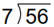2-digit divided by 1-digit, no remainder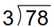2-digit divided by 1-digit, remainder possible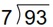3-digit divided by 1-digit, no remainder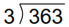3-digit divided by 1-digit, remainder possible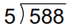4-digit divided by 1-digit, no remainder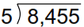4-digit divided by 1-digit, remainder possible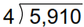Sample Grade 4 Long Division Worksheet

## More division worksheets

Explore all of our division worksheets, from simple division facts to long division of large numbers.

What is K5?

K5 Learning offers free worksheets, flashcards and inexpensive workbooks for kids in kindergarten to grade 5. Become a member to access additional content and skip ads.